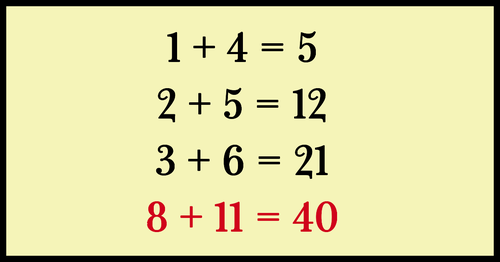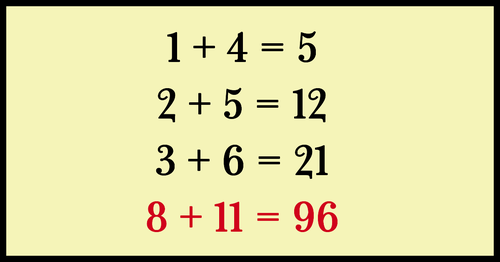# Warning: only people with iqs of 130+ can pass this insane viral math puzzle

This is a topic of interpretation, the puzzle could have many possible answers, but so far we have two possible solutions. This will look hard at first, but the solutions explain it all step by step.

Above is a math problem which you need to solve, however, there are two possible solutions both which are illustrated below. First, try and get your head around the first solution before attempting the next one. If you get stuck, you can scroll down to check out the answer. Let us know which one you worked out first in comments. Good luck!Solution 1 explained:

Most agree that 1 + 4 = 5.
In the next line, add 2 + 5 to the sum of 5 in the equation above. Total = 12
Next, apply the same formula: 3 + 6 = 9, then add the 9 to the sum in the equation above (12). Total = 21.
The last step is to take the 8 + 11= 19 and add it to the sum of the previous problem (21). Total = 40.
Try again for the second solution!Solution 2 explained:

The obvious truth is: 1 + 4 = 5. But you could also reach 5 by adding 1 to 4 times 1.
In the same fashion, with the second line, 2 + 2(5) = 12.
And the next line: 3 + 3(6) = 21.
Using this method to solve the problem, 8 + 8 x 11 = 96.
Which answer did you get? 40 or 96?

If you liked this math puzzle, hit that Liked Video on Facebook button to challenge your friends and loved ones 🙂

Source

1